#### WATCH ALL SLIDES

Slide 1Waves

This PowerPoint Presentation is intended for use during lessons to match the content of Waves and Our Universe - Nelson

Either for initial teaching

Or for summary and revision

Slide 2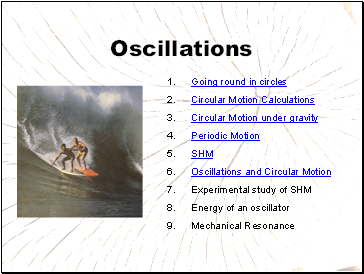## Oscillations

Going round in circles

Circular Motion Calculations

Circular Motion under gravity

Periodic Motion

SHM

Oscillations and Circular Motion

Experimental study of SHM

Energy of an oscillator

Mechanical Resonance

Slide 3## Waves

Travelling waves

Transverse and Longitudinal waves

Wave speed, wavelength and frequency

Bending Rays

Superposition

Two-source superposition

Superposition of light

Stationary waves

Slide 4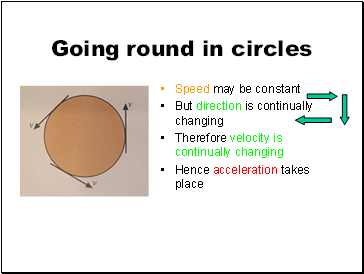## Going round in circles

Speed may be constant

But direction is continually changing

Therefore velocity is continually changing

Hence acceleration takes place

Slide 5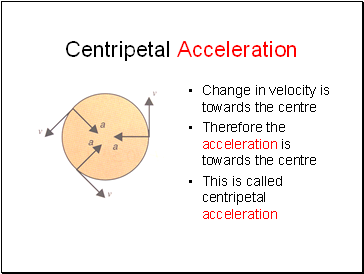## Centripetal Acceleration

Change in velocity is towards the centre

Therefore the acceleration is towards the centre

This is called centripetal acceleration

Slide 6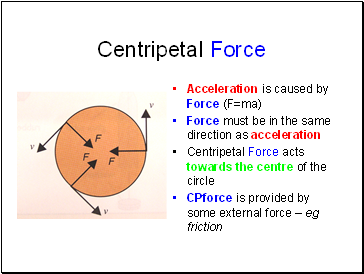## Centripetal Force

Acceleration is caused by Force (F=ma)

Force must be in the same direction as acceleration

Centripetal Force acts towards the centre of the circle

CPforce is provided by some external force – eg friction

Slide 7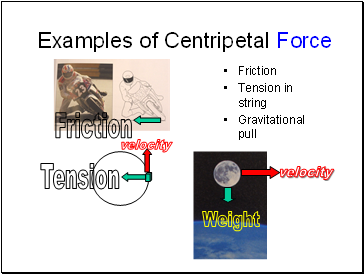## Examples of Centripetal Force

Friction

Tension in string

Gravitational pull

Friction

Tension

Weight

velocity

velocity

Slide 8Centripetal Force 2

What provides the cpforce in each case ?

Slide 9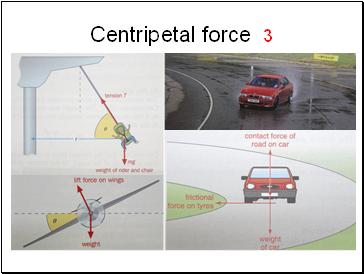Centripetal force 3

Slide 10## Circular Motion Calculations

Centripetal acceleration

Centripetal force

Slide 11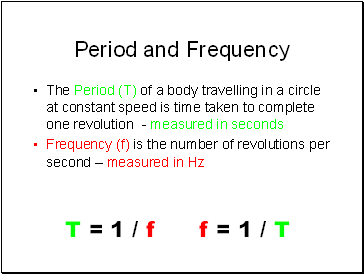## Period and Frequency

The Period (T) of a body travelling in a circle at constant speed is time taken to complete one revolution - measured in seconds

Frequency (f) is the number of revolutions per second – measured in Hz

T = 1 / f f = 1 / T

Go to page:
1  2  3